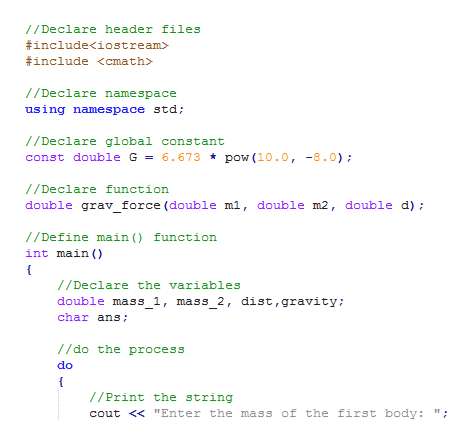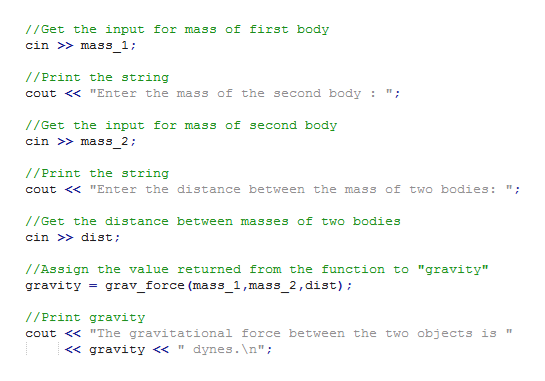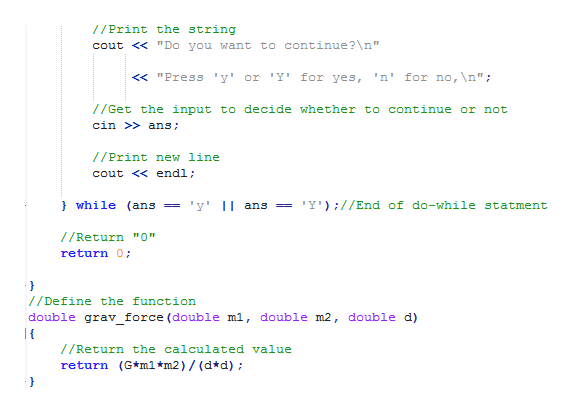# m2 separated by a distance d is given by:F = Gm1m2d 2where G is the universal gravitational constant:G = 6.673 × 10−8 cm3g × sec2Write a function definition that takes arguments for the masses of two bod-ies and the distance between them and that returns the gravitational force. Since you will use the preceding formula, the gravitational force will be in dynes. One dyne equalsg × cmsec2You should use a globally defined constant for the universal gravitational constant. Embed your function definition in a complete program that computes the gravitational force between two objects given suitable inputs. Your program should allow the user to repeat this calculation as often as the user wishes. C++

Question
32 views

m2 separated by a distance d is given by:
F = Gm1m2
d 2
where G is the universal gravitational constant:
G = 6.673 × 10−8 cm3
g × sec2
Write a function definition that takes arguments for the masses of two bod-
ies and the distance between them and that returns the gravitational force.
Since you will use the preceding formula, the gravitational force will be in
dynes. One dyne equals
g × cm
sec2

You should use a globally defined constant for the universal gravitational
constant. Embed your function definition in a complete program that
computes the gravitational force between two objects given suitable inputs.
Your program should allow the user to repeat this calculation as often as
the user wishes.

C++

check_circle

Step 1

A C++  program to display the gravitational force between two objects is given below;

Program code:

#include<iostream>

#include <cmath>

//Declare namespace

using namespace std;

//Declare global constant

const double G = 6.673 * pow(10.0, -8.0);

//Declare function

double grav_force(double m1, double m2, double d);

//Define main() function

int main()

{

//Declare the variables

double mass_1, mass_2, dist, gravity;

char ans;

//do the process

do

{

//Print the string

cout << "Enter the mass of the first body: ";

//Get the input for mass of first body

cin >> mass_1;

//Print the string

cout << "Enter the mass of the second body : ";

//Get the input for mass of second body

cin >> mass_2;

//Print the string

cout << "Enter the distance between the mass of two bodies: ";

//Get the distance between masses of two bodies

cin >> dist;

/*Assign the value returned from the function to

"gravity" */

gravity = grav_force(mass_1,mass_2,dist);

//Print gravity

cout << "The gravitational force between the two objects is "<< gravity << " dynes.\n";

//Print the string

cout << "Do you want to continue?\n"

<< "Press 'y' or 'Y' for yes, 'n' for no,\n";

//Get the input to decide whether to continue or not

cin >> ans;

//Print new line

cout << endl;

} while (ans == 'y' || ans == 'Y');//End of do-while statement

//Return "0"

return 0;

}

//Define the function

double grav_force(double m1, double m2, double d)

{

//Return the calculated value

return (G*m1*m2)/(d*d);

}

Screenshot of program #1:help_outlineImage TranscriptioncloseDeclare header files #include //Declare namespace using namespace std; Declare global constant const double G= 6.673 * pow (10.0, -8.0) //Declare function double grav_force (double ml, double m2, double d); //Define main () function int main f /Declare the variables double mass_1, mass_2, dist, gravity; char ans //do the process do f /Print the string cout <"Enter the mass of the first body: fullscreen
Step 2

Screenshot of program #2:help_outlineImage Transcriptionclose1/Get the input for mass of first body cin >mass 1 //Print the string cout <"Enter the mass of the second body: //Get the input for mass of second body cin >mass 2; //Print the string cout <"Enter the distance between the mass of two bodies: //Get the distance between masses of two bodies cin dist //Assign the value returned from the function to "gravity" grav force (mass 1,mass 2,dist); gravity //Print gravity cout <"The gravitational force between the two objects is dynes.\n"; < gravity << " fullscreen
Step 3

Screenshot of program #3...help_outlineImage Transcriptionclose//Print the string cout <" Do you want to continue?\n" 'n' for no, \n" y' or Y' for yes, <"Press /Get the input to decide whether to continue or not cin >ans //Print new line cout < endl; }while (ans 'Y') ;//End of do-while statment y ans //Return "0" return 0; //Define the function double grav force (double ml, double m2, double d) /Return the calculated value return (G*m1*m2)/ (d*d) } fullscreen

### Want to see the full answer?

See Solution

#### Want to see this answer and more?

Solutions are written by subject experts who are available 24/7. Questions are typically answered within 1 hour.*

See Solution
*Response times may vary by subject and question.
Tagged in

### Programing Language